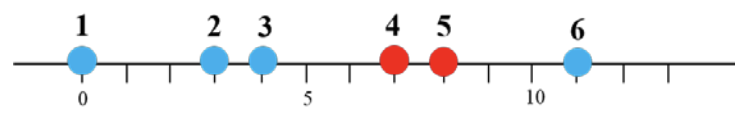시간 제한메모리 제한제출정답맞힌 사람정답 비율
0.5 초 512 MB270805926.457%

## 문제

There are n distinct points with m colors on the line, where m ≤ n. Let S be the set of those points. We want to select a non-empty subset CS that satisfies the following:

For every point p in S - C, not belonging to C, the closest point of p among points in C has the same color as p. Of course, if there are more than one closest point of p in C, then it is sufficient that one of them has the same color as p.

For example, there are six points labeled by 1 to 6 with two colors in Figure G.1. Points 4 and 5 are red and the others blue. The set {2, 4, 6} satisfies the above property. But the set {2, 4} does not satisfy the property, because the closest point of point 6 in {2, 4} is point 4, which is different from the color of point 6. In fact, the set {2, 4, 6} is a minimum cardinality subset to satisfy the property.Figure G.1: Six colored points on a line

Given n distinct points on the line and m their colors, write a program to find a non-empty subset C of S with minimum cardinality satisfying the above property and to output the minimum cardinality.

## 입력

Your program is to read from standard input. The input starts with a line containing two integers, m and n (1 ≤ mn ≤ 100,000), where m is the number of colors and n is the number of points. The points are numbered 1 to n from left to right on the line, and the colors are numbered 1 to m. The second line contains a sequence of sorted n integers in increasing manner, where the i-th number is the coordinate of the point i. The coordinates x of points satisfy 0 ≤ x ≤ 109 and are all distinct. The third line contains a sequence of n integers, where the i-th number is the color of the point i, which is between 1 and m.

## 출력

Your program is to write to standard output. Print exactly one line. The line should contain the minimum cardinality of a non-empty subset C of S to satisfy the above property.

## 예제 입력 1

2 6
0 3 4 7 8 11
1 1 1 2 2 1


## 예제 출력 1

3


## 예제 입력 2

2 6
0 3 4 7 8 11
1 2 1 2 2 1


## 예제 출력 2

5